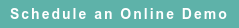# RoyaltyStat Blog

### Posts by Topic

Computation of an arm's length operating profit margin is not as easy as it seems. As an example, we consider the directly proportional profit model postulated by the OECD Transfer Pricing Guidelines and the more likely power function that we may encounter when we analyze actual comparable data including small and large enterprises. In cases when the selected comparables include small, medium and large uncontrolled enterprises, an arm's length operating profit margin is more likely to be reliably measured by a double log or power function. The operating profit margin is defined as operating profits (before or after depreciation and amortization) divided by sales revenue.

No Intercept Linear Profit Function

The OECD TNMM (CPM in the U.S.) prescribes a naïve model between “net” (operating) profits (Y) and sales (X), denominated in a specific currency:

(1)     Yi = β Xi for i = 1, 2, …, N comparable enterprises

where β is the estimated “net” (operating) profit margin. We call this model naïve because it provides that in general “net” profits are directly proportional to sales, i.e., this model contains no explanatory factor after deflation of equation (1) by X.

To simplify exposition, we set aside the random error that must be added to equation (1), which allows for the computation of a statistical interval around the slope coefficient. The OECD narrative of the TNMM does not define “net” profits using IFRS terminology, and it’s an obvious solecism when “net” profits appears to mean operating profits before or after a depreciation deduction. See OECD 2010 Guidelines, ¶¶ 2.48, 2.58 and the Glossary: “Net profit indicator: The ratio of net profit to an appropriate base (e.g. costs, sales, assets). The transactional net margin method [TNMM] relies on a comparison of an appropriate net profit indicator for the controlled transaction with the same net profit indicator in comparable uncontrolled transactions.”

Log-Linear Profit Function

Instead of equation (1), operating profits and sales may produce the best statistical curve fit by using a power function:

(2)     Yi = α Xβ

where the “net” (operating) profit margin is the slope coefficient {β (Yi /Xi)} of equation (2), instead of β alone, as naively determined by equation (1). This power function states that Yi is proportional to Xiβ, i.e., operating profits are determined by sales revenue elevated to the power of beta. We get significantly different results using model (1) versus (2) when the estimate of beta from equation (2) diverges from 1.0, such as when the estimate of the beta coefficient is 0.9 or 1.2.

Slope Coefficient

The slope coefficient of equation (2) is the derivative of Y (operating profits) with respect to X (sales revenue):

(3)     Yi’ = α β Xi β – 1

were Yi (with an apostrophe) denotes the first derivative.

We can simplify equation (3) by getting rid of the exponent. First, we make use of two exponent rules in algebra (1 / X = X−1) and (X/ Xn = Xmn), and obtain an intermediate expression of the slope coefficient of this power function:

(4)     Yi’ = (α β Xi β / Xi)

Second, we substitute for Y from equation (2), and obtain a simple expression of the slope coefficient without an exponent:

(5)     Yi’ = β (Yi / Xi)

In equation (5), the β coefficient is weighted (multiplied) by a comparable profit margin (Yi / Xi). It’s sensible to use the comparable median or the average (free of outliers) operating profit margin as a multiplicative constant of β to determine the arm’s length profit margin of the “tested party” (controlled taxpayer) when a power function is a better fit than the OECD postulated linear function.

In general, the intercept and slope coefficient of equation (2) are estimated using regression analysis by taking the natural logarithms of both sides to obtain a double log regression function:

(6)     LN(Yi) = LN(α) + β LN(Xi)

We can also compute the slope = β (Yi / Xi) using Excel. E.g., starting with the Y (operating profits) and X (sales revenue) data columns of the selected comparables, we create two additional columns LN(Y) and LN(X). Next, we use the Excel internal function SLOPE = (LN(Y), LN(X)). As a final step, we multiply the calculated slope by the median or the average (free of outliers) (Y / X) in order to obtain β (Yi / Xi).

Published on Sep 2, 2016 12:22:00 PM

Ednaldo Silva (Ph.D.) is founder and managing director of RoyaltyStat. He helped draft the US transfer pricing regulations and developed the comparable profits method called TNNM by the OECD. He can be contacted at: esilva@royaltystat.com

RoyaltyStat provides premier online databases of royalty rates extracted from unredacted license agreements
and normalized company financials (income statement, balance sheet, cash flow). We provide high-quality data, built-in analytical tools, customer training and attentive technical support.Topics: Net profit